My first try at MLflow

tl;dl MLflow is cool but still requires some work to use it.

Imagine that several data scientists work for a data science project. They have to train a good predictive model. They have a lots of ideas. Thus they process the row data in various ways, sometimes add an additional data source to the original data, select features to remove non-significant features, and try to train various models, maybe including a multi-layer neural network. They do lots of experiments in order to improve their model.

At the end they have to deliver the best model: the feature matrix can be constructed by converting data in a certain method and we train a neural network with 4 layers. But where is the source code? Because they work Jupyter Notebook, there is an exported HTML but no executable script? Ok, I found it... But wait, the result is different from the previous result in the exported HTML file. Why?

Data science is science. We do lots of experiments to obtain a good predictive model. But if we do not manage trained models carefully, we will face a problem of reproducibility.

So how can we manage the trained models? Take a note about each model? Git commit with the result? Well, in fact there are several applications and web services for that purpose. For example FLOYDHUB and comet. They looks good but it is quite expensive for a small team.

Therefore I tried MLflow as a substitute of you.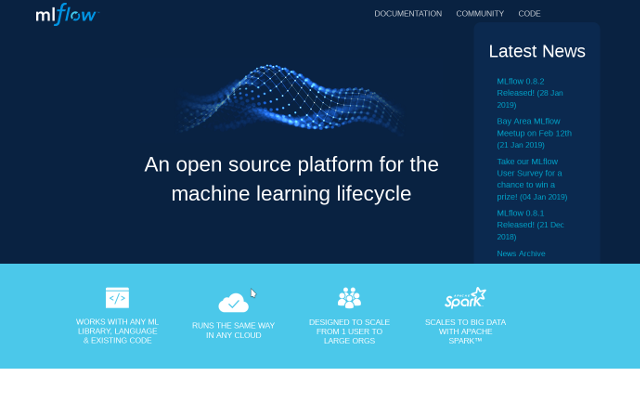MLflow is an open source project which is developed mainly by Databricks. The application has already supported many machine learning libraries/frameworks: scikit-learn, PyTorch, TensorFlow, Apache Spark. You can also use the application on R. According to the documentation, we do not need to learn not so many new things. Why don't we try it?

## Too short overview of MLflow

It is definitely better to watch an official presentation for MLflow.

Roughly speaking, MLflow consists of three components:

• Tracking: logger of training
• Projects: definition of the environment (conda env + how to execute)
• Models: web API of the trained model

MLflow Tracking logs a commit hash so that you can find the source code which produces a specified result.

MLflow Projects is just a specification of a ML pipeline. A text file MLproject describes which development environment is used and how a script must be executed. Maybe you can easily understand it if you see an example.

MLflow Models provides an easy way to provide a trained model as a web API.

So in the best scenario you can train many models you like, after that you can chose the best model from MLflow Tracking and thanks to MLflow Projects, you can reproduce the result. Moreover it is easy to integrate the trained model in a production system as part of microservices.

Yes, it sounds really cool.

## Here is a data science project

WARNING

• My usage of MLflow is probably different from what the MLflow developers expect.
• The version of MLflow is 0.8.1. Not the newest.

You have a large database. Every hour, every minute, every second you receive many records such as user behaviour on a web site. You want to create a better model which provides a better result for a business problem. So you want to give a new and better model regularly. The new model should be provided as a REST API, so that it is easy to apply the trained model from another server. Since the data is quite large and the project is very important for the business, several data scientists work for the project.

Can you imagine the project well?

Then let us consider what we have to do.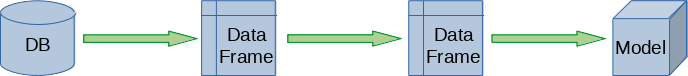As you see there are three steps.

2. Processing: Convert the collected data into a single feature matrix.
3. Training: Train a mathematical model with a machine learning algorithm.

Each step can be done by a different data scientist. (I do not mean that a data scientist is responsible only for one of the steps.) So each script belongs to one of the steps. Thus in order to execute the whole pipeline we have to execute the three scripts in the right order after activating the environment we need.

$conda activate yourenv$ python load-data.py     ## Loading
$python fs_stats_test.py ## Processing$ python randomforest.py  ## Training


MLflow Projects enables us to package the whole procedure. If you write MLproject then the following command gets the job done.

$mlflow run .  Since the last script stores the trained model, we can easily provide the trained model through web API $ mlflow pyfunc serve -p 1234 -m ....


It's easy, isn't it?

## Challenges

Well, in fact, it is not so easy. We have to care about lots of things. In other words you need to prepare several helper functions/classes to integrate the project into MLflow.

The challenges which I am going to say can be applied for another similar machine learning project framework.

### 1. Coherent chain of steps

MLflow Tracking logs the commit hash when you submit the results (parameters and metrics) automatically. So you can obtain the script which produces the needed result.

Yeah, you might forget to commit the modification before starting a run. (I will explain later what a run is.) This might be a typical mistake, but we ignore this: Everybody commits before starting a run.

But the problem is: A different script is based on a different commit.Imagine that a data scientist writes a script for data processing and start several runs. The first run fills missing values by the mean value. The second run fills the missing values by a random forest model. The third run adds an additional column which can be useful for us. The fourth run selects several columns by using a statistical test.

You can convert the data into a feature matrix in several ways and this is one of the important steps to train a good model. And the best feature matrix depends on a model and data. Last week the best model was a logistic regression with a feature matrix whose missing values were filled by a random forest. Because you got a lots of new data, the best model of this week can be an XGB model with a feature matrix whose missing values are filled by the mean value. This can really happen.

It is best that several feature matrices are available at any time. But every branch has only one direction, not several. Thus we have to manage several data processing somehow.

### 2. Data-versioning

A data scientist fetches data, say D1, and trains a model, say M1. Next week a different data scientist fetches data, say D2, and trains a different model, say M2. Then it is nonsense to compare CV scores of M1 and M2.

(If we train the same model on D1 and D2, then you looks something similar to the learning curve, but it is not the case.)

Data has also a version. In one ML life cycle the data version should not changes. But it can change between two cycles.

Problem is: the version of the script does not correspond to the version of the data. The same script can fetch different data set. Namely a commit hash does not help us. We have to manage the versions of data in a suitable way.

### 3. Input data to the API

Using MLflow Modells we can easily store the trained model.

mlflow.sklearn.log_model(model, "model")


And we can provide the Web API of the trained model very easily. But the input data must suit the features in the feature variables. In other words, you have to apply data processing in advance.

If you want to use MLflow Modell in a production system, you cannot assume that the input data has already been processed as you expect. As we said above, the data processing can also change. Therefore you have to integrate your data processing between the raw data and the web API of MLflow.

## Possible solutions

In my opinion one of the easy possible solutions to the challenges 1 and 2 is to use parameters.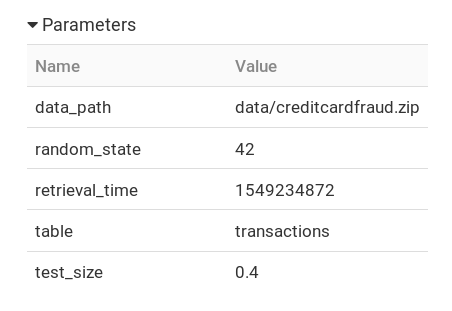Before going into details, we would like to explain some important features and concepts for MLflow Tracking very briefly.

A run is a unit of what you to want to log. A typical example is a model training.

with mlflow.start_run():
### this and that ..
mlflow.log_param("algorithm", "LogisticRegression")
mlflow.log_param("C", 10)
mlflow.log_metric("training score", 0.890)
mlflow.log_metric("test score", 0.789)


Then parameters and metrics are logged as the result of the run. The commit hash of the script is also logged. (Thus you can find the source code which you executed.) We should note that an option of the script is regarded as a parameter. Namely

$python main.py --table transactions  is same as mlflow.log_param("table", "transactions")  This is technical, but we need to care about it when we design a process with MLflow. ### Data-Versioning First we consider the data-versioning. You should give two parameters: • Type of data: the name of a table or a query • Retrieval time: when you retrieved the data The pair of the two is an ID of the data or a run. Just it. Your query is something like select * from t where year = '2018', and therefore you think you should use year as a parameter instead of the retrieval time? No, you should use it as an additional parameter, but it is better to have retrieval time. That is because the query can change. What if the query select * from t where year = '2018'  becomes select t.*, s.col1 from t left join s on t.id = s.id where t.year = '2018';  because you need more data? Both queries return a data for 2018. ### Coherent chain of steps Actually we can tackle the first challenge in the same way. That is, we use a pair of "type" and executed time (run_time) as an ID of each step.  step type run_time loading table loaded_time processing logic processed_time training algorithm trained_time Consider you write a script for data processing. Then your logic (how to fill missing values, how to create a new variable, etc.) is of course based on the data. Therefore a run of your data processing must have the ID of the run for the data loading and you also give the pair of logic (short name for the data processing) and processing_time. Therefore a run for data processing contains (at least) the following • table (query of the run for load) • retrieval_time (run time of the load run) • logic (query of the processing run) • processing_time (run time of the processing) The first two parameters specify the run for data load and the pair of the third and fourth parameters is the ID of the processing run.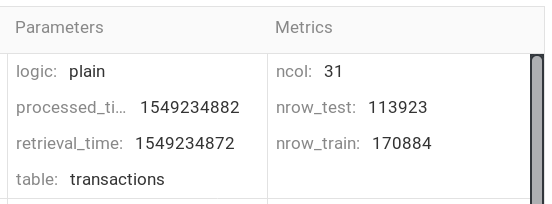We can apply the same idea to a run in "training". That is, we give the ID of the training run (algorithm and trained_time) and the ID of the processing run which the training run is based on. (Of course you should give more parameters such as values of hyperparameters.)### Input data to the API This is a relatively technical problem. At first I naïvely thought that it is OK to use mlflow.py_func. But I do not still understand how we can make use of this module for our purpose. So I use a trick. The basic idea is easy to understand. If we can store an instance of the following class, then you can use it as "model". class MyModell: def __init__(self, processor, model): self.processor = processor self.model = model def predict(self, X, y=None): X_processed = self.processor(X) return self.model.predict(X_processed)  I wrote a complete version of the class. You can store an instance of the class by mlflow.sklearn.log_model processor is a function which converts a row data into a feature matrix. It typically has an internal state such as a fitted LabelBinalizer. You store the function as an artifact at the processing run. They are two problems: 1) If you save a function with pickle, then you might not be able to deserialise the pickled function. In such a case you need to use dill instead of pickle. 2) MyModell class must be installed on the conda environment. Otherwise you can not deserialise the logged model. This is the reason why I wrote a simple class and put it on GitHub. You can install the module by the command $ pip install https://github.com/stdiff/model_enhanced/archive/v0.2.zip


In conda.yaml you can simply put

- https://github.com/stdiff/model_enhanced/archive/v0.2.zip


## Implementation of a ML project

MLflow is a flexible framework. This is important because a process of a data science project normally depends largely on the project itself.

But in my opinion lots of functions still lack, especially ones which can deal with runs in an easy way. Therefore I have developed a sample project: mlflow-app.

Warning:

• The convention is slightly different from this blog entry. This is because I changed lots of concepts during development.
• My implementation is incomplete. There is no unit test, several values are hard-coded, it can not easily extend to a general project process.
• That is, this application is just a PoC, not of a productive quality.

I assume that Anaconda is installed. You can clone the source code from my repository by the following command

git clone git@github.com:stdiff/mlflow-app.git


Then you have to download the data set: Credit Card Fraud Detection. Put the zip file under data directory. We are going to build a predictive model for anomaly detection.

Next you need to create a conda environment and activate it:

$conda env create -n pipeline --file conda.yaml$ conda activate pipeline


Now MLflow is available in the environment. Start the MLflow UI on the port 5009.

(pipeline) $mlflow ui --port 5009 &  There is no specific reason for 5009. But if you want to change the port number then you have to change "config.ini" accordingly. Then we create three experiments. Here an experiment is a set of runs. When you start a run, you can specify an experiment which the run should belong to. (pipeline)$ MLFLOW_TRACKING_URI=http://localhost:5009 mlflow experiments create load
Created experiment 'load' with id 1

(pipeline) $MLFLOW_TRACKING_URI=http://localhost:5009 mlflow experiments create processing Created experiment 'processing' with id 2 (pipeline)$ MLFLOW_TRACKING_URI=http://localhost:5009 mlflow experiments create model
Created experiment 'model' with id 3


The id numbers are important, because we use them when starting a run.

(pipeline) $mlflow run --no-conda -e load --experiment-id 1 . -P table=transactions  This command execute load/load_data.py which splits the whole data into a training set and a test set. The result (the training set and the test set) of this run is logged as "artifacts" in experiment "load". After the execution you can check the result from the Web UI. The following command starts a run for data processing. It converts the training set and the test set in a certain way and stores the function for the data processing as an artifact. Change the retrieval_time before executing it. (pipeline)$ mlflow run --no-conda -e processing --experiment-id 2 . -P table=transactions -P retrieval_time=2019-02-09


The last step is a hyperparameter tuning with GridSearchCV. The model is the (penalised) logistic regression. Do not forget to change processed_time.

(pipeline) $mlflow run --no-conda -e model --experiment-id 3 . -P logic=plain -P processed_time=2019-02-09  This process takes very long (around 1 hour) so, if you want to avoid such a heavy training for PoC add the following line to line 152. df = df.head(10000)  The trained model is saved as a "model" and you can start easily the web API of the model. From the web UI you need to check the path to the model, then execute the following command with the path to the model (pipeline)$ mlflow pyfunc serve -p 1234 --no-conda -m /xxxxxxx/artifacts/model


Then http://127.0.0.1:1234/invocations will be the endpoint of the API.

Finally the coherent pass of steps is checked by main.py.

(pipeline) $mlflow run --no-conda -e main . -P retrieval_time=2019-02-09 -P table=transactions -P logic=plain -P algorithm=LogisticRegression  This command starts the given run for data loading (table, retrieval_time) and then look for a newest coherent path within the given types (logic, algorithm). If there is no coherent path, then a run is started. The coherent path can be found in experiment "Default".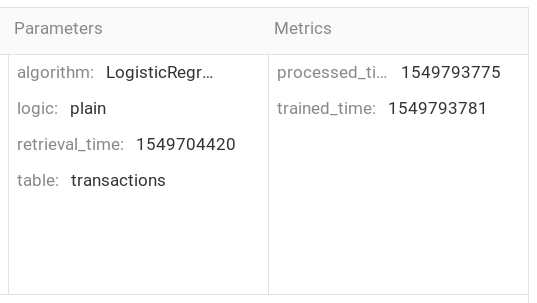In this case the run for training with (LogisticRegression, 1549793781) is the last step and stores the trained model you need. ### Implementation is not so easy Large part of the source codes is for MLflow while the analysis itself is easy. You can find the corresponding analysis in this notebook. If you would like just to log the result of the training, then it is easy to use MLflow (Tracking). But If you want to integrate MLflow into the project process which we discussed above, then you need to write several helper functions and a small class. This is because MLflow does not provide functions to search a run. I needed to write functions for that purpose. ### FAQ for my implementation #### Why don't we use run id? Do you want to deal with a string like "20128fa478b4458e945260a124b72c4f"? If yes, use it. But if you do so, then you can not make use of "type" of a run. That is, "searching the newest data processing by a certain logic" becomes nonsense. #### Why do we use unix time? For the main entry point we give processed_time and trained_time as metrics. MLflow requires that a metric is a number, therefore we can not use an ordinary time description. If you want to use a more user-friendly description, there are (at least) two solutions. 1. Use "yyyymmddHHMMSS" format (in UTC), then you can use the description as a parameter (str) and a metric (int) at the same time. 2. Stick with parameters. (Do not give a time description in "metric"). #### Why don't we use start time? The reason is the same as above. #### But we use a date when starting a run. If a date is given, then we look for the newest run within the given date. #### Why do we do "train-test-splitting" in data loading. You don't need to do it in data loading. PoC is just an example. #### What if there are two data sources? Imagine there two tables you need. Then the easiest solution would be to fetch the two tables in one run and store them as artifacts. Another solution would be to implement logic so that we can specify two runs for data loading. (E.g. table=transactions,customers.) #### Why don't we extend the "multistep_workflow" example? You can find the example "multistep_workflow" in the MLflow repository. There are two points I don't like. • The whole steps belong to one experiment. This makes it difficult to compare runs in the same steps. • A dependency chain is not clear. #### Why don't we make use of conda at the run time? If several data scientists work for a project, then the development environment should be unified. That is, there should be conda.yaml which is managed by the project team. Then why don't we use it? ### Further Development The implementation in the GitHub is just a minimal PoC. And actually I have not implemented several things. I write them. #### Modularisation of a type. The type should be specified by a parameter. That is, if we execute the following command $ mlflow run -e model . -P algorithm=rf [...]


then a code for random forest model should be executed,

\$ mlflow run -e model . -P algorithm=xgboost [...]


then a code for XGBoost model should be executed. Namely the script for the entry point of training is unique and the algorithm should be chosen by a parameter. We need to write something like the following:

if algorithm == "rf":
result = rf(data, parameters)
elif algorithm == "xgboost":
result = xgboost(data, parameters)


The functions such as rf or xgboost are defined in a separate script.

I wrote several functions and codes for searching a specified run. The interface should be more unified and we should be able to use without knowing the details of the classes of MLflow.

Of course.

## Problems

I find MLflow very cool, but it is still a beta release. There are several things to do before the official release.

### Documentation

I have not understood relations among the classes of MLflow yet. For example lots of classes related to a run belong to mlflow.entities. Here is a question: How can we get all key-value pairs of parameters of a run with a given run id?

1. Get the experiment id with a MLflow client instance.
2. Get the list of RunInfo instances with client.list_run_infos(experiment_id).
3. Each RunInfo instance has data property with the value in RunData.
4. RunData instance has an attribute param which is a list of Param instances.
5. Each Param instance has key and value properties. All pairs of the properties are what we want.

Well, it is very difficult to find/understand these relations from the documentation.

(To be honest I don't like Java-style-OOP like this. For example an Experiment instance has no information about runs which belong to the experiment. Experiment class is used only to keep very small piece of information.)

I strongly recommend to write docstring in detail.

class Run(_MLflowObject):
"""
Run object.
"""


This docstring is not helpful at all.

### Deletion of the directory of a run

If a run is removed, then the related files should also be removed. It is very difficult to remove directories of the removed runs manually because the directory name of a run is run id.

### Experiment name instead of id

An experiment name should be available when executing a mlflow run command.

### Don't ignore set_experiment method

If we start a run by "mlflow run" command, then the setter

mlflow.set_experiment("your_special_experiment")


is ignored. I don't think that it is a good behaviour.

## Summary

• MLflow is a useful application/framework to manage a data science project.
• For a simple usage MLflow works out of the box.
• If you need a non-simple usage, then you have to implement several functions/classes.
Categories: #data-mining